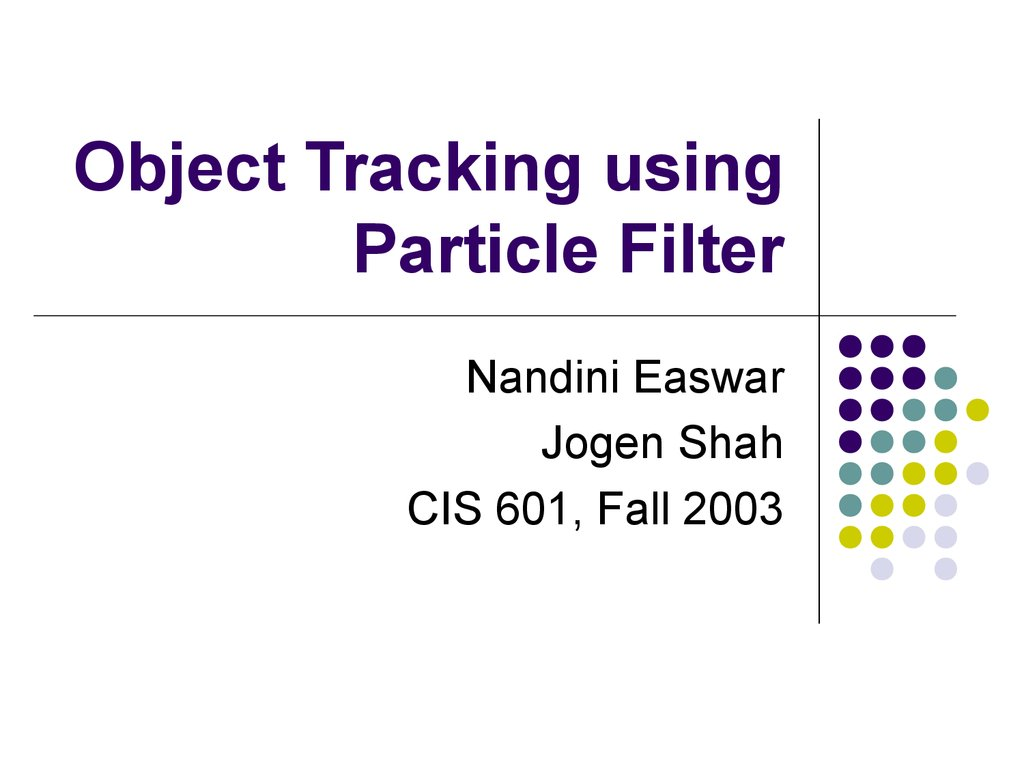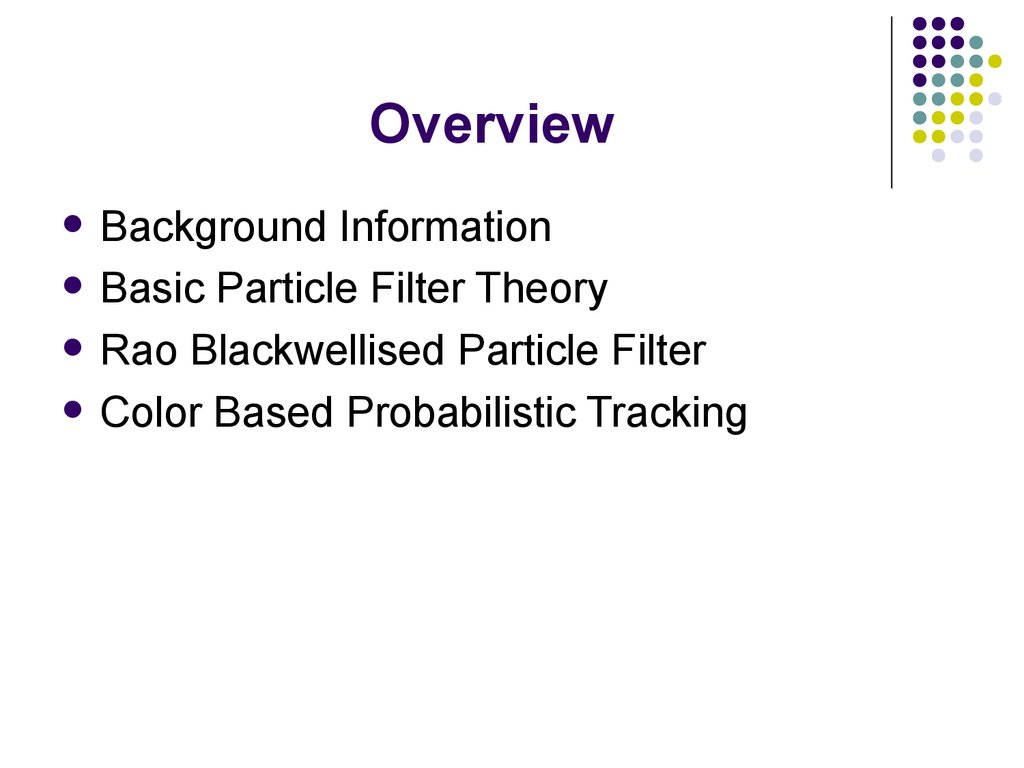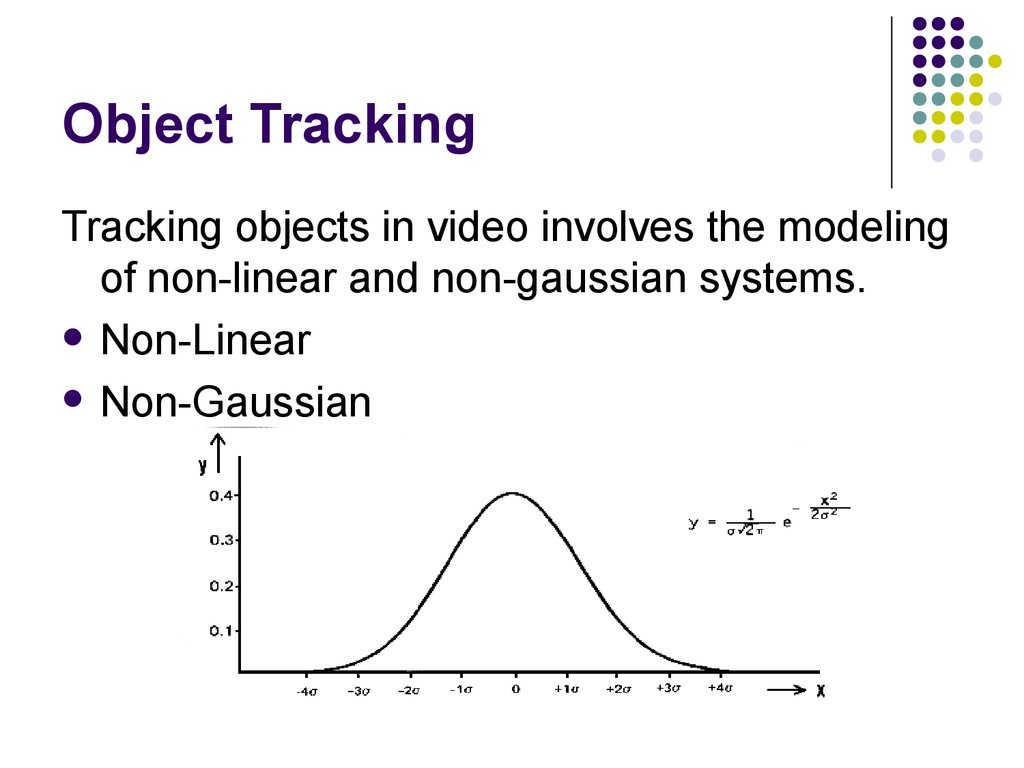# Object tracking using particle filter

## 1. Object Tracking using Particle Filter

Nandini Easwar
Jogen Shah
CIS 601, Fall 2003

## 2. Overview

Background Information
Basic Particle Filter Theory
Rao Blackwellised Particle Filter
Color Based Probabilistic Tracking

## 3. Object Tracking

Tracking objects in video involves the modeling
of non-linear and non-gaussian systems.
Non-Linear
Non-Gaussian

## 4. Background

In order to model accurately the underlying
dynamics of a physical system, it is important
to include elements of non-linearity and
non-gaussianity in many application areas.
Particle Filters can be used to achieve this.
They are sequential Monte Carlo methods
based on point mass representations of
probability densities, which are applied to any
state model.

## 5. The Particle Filter

Particle Filter is concerned with the problem
of tracking single and multiple objects.
Particle Filter is a hypothesis tracker, that
approximates the filtered posterior distribution
by a set of weighted particles.
It weights particles based on a likelihood
score and then propagates these particles
according to a motion model.

## 6. Mathematical Background

Particle Filtering estimates the state of the
system, x t, as time t as the Posterior
distribution:
P( x t | y 0-t )
Let,
Est (t) = P( x t | y 0-t )
Est(1) can be initialized using prior
knowledge

## 7. Mathematical Background

Particle filtering assumes a Markov Model for
system state estimation.
Markov model states that past and future
states are conditionally independent given
current state.
Thus, observations are dependent only on
current state.

## 8. Mathematical Background

Est(t) = P( x t | y 0 - t )
= p(y t | x t, y 0 – t-1).P(x t | y 0 – t-1)
(Using Baye’s Theorem)
= p(y t | x t ). P(x t | y 0 – t-1)
(Using Markov model)
= p(y t | x t ). P(x t |x t-1).P(x t-1 | y 0 – t-1)
= p(y t | x t ). P(x t |x t-1).Est(t-1)

## 9. Mathematical Background

Final Result:
Est(t) = p(y t | x t ). P(x t |x t-1).Est(t-1)
Where:
p(y t | x t ): Observation Model
P(x t |x t-1).Est(t-1): Proposal distribution

## 10. Mathematical Background

To implement Particle Filter we need
State Motion model: P(x t |x t-1)
Observation Model: p(y t | x t ):
Initial State: Est(1)

## 11. Mathematical Background

We sample from the proposal and not the posterior
for estimation.
To take into account that we will be sampling from
wrong distribution, the samples have to be likelihood
weighed by ratio of posterior and proposal
distribution:
W t = Posterior i.e.Est (t) / proposal Distribution
= p(y t | x t )
Thus, weight of particle should be changed
depending on observation for current frame.

## 12. Basic Particle Filter Theory

A discrete set of samples or particles
represents the object-state and evolves over
time driven by the means of "survival of the
fittest". Nonlinear motion models can be used
to predict object-states.

## 13. Basic Particle Filter Theory (Cont.)

Particle Filter is concerned with the
estimation of the distribution of a stochastic
process at any time instant, given some
partial information up to that time.
The basic model usually consists of a Markov
chain X and a possibly nonlinear observation
Y with observational noise V independent of
the signal X.

## 14. Basic Particle Filter Theory (Cont.)

System Dynamics ie.Motion Model:
p(x t| x 0:t-1)
Observation Model:
p(y t | x t)
Posterior Distribution:
p(x t | y o..t)
Proposal Distribution is the Motion Model
Weight, w t = Posterior / Proposal = observation

## 15. Basic Particle Filter Theory (Cont.)

Given N particles (samples)
{x(i)0:t-1,z(i)0:t-1}Ni=1 at time t-1,
approximately
distributed according to the distribution
P(dx(i)0:t-1,z(i)0:t-1|y1:t-1), particle filters enable us
to compute N particles {x(i)0:t,z(i)0:t}Ni=1
approximately distributed according to the
posterior distribution P(dx(i)0:t,z(i)0:t|y1:t)

## 16. Basic Particle Filter Theory (Cont.)

The basic Particle Filter algorithm consists of
2 steps:
Sequential importance sampling step
Selection step

## 17. Particle Filter Algorithm

Sequential importance sampling
Uses Sequential Monte Carlo simulation.
For each particle at time t, we sample from the
transition priors
For each particle we then evaluate and normalize the
importance weights.

## 18. Particle Filter Algorithm

Selection Step
Multiply or discard particles with respect to high or
low importance weights w(i)t to obtain N particles.
This selection step is what allows us to track
moving objects efficiently.

## 19. Rao-Blackwellised Particle Filter

RBPF is an extension on PF.
It uses PF to compute the distribution of
discrete state with Kalman Filter to compute
the distribution of continuous state.
For each sample of the discrete states, the
mean and covariance of the continuous state
are updated using the exact computations.
We have implemented the particle filter
algorithm and not the RBPF.

## 20. RBPF Approach

RBPF models the states as <Ct,Dt>
Ct is the continuous state representation
Dt is the discrete state representation
The aim of this approach is to predict the
discrete state Dt.
However, for our object tracking application,
the above approach was unsuitable.

## 21. Implementation

We have implemented the Particle Filter
algorithm in Matlab.
Our approach towards this project:
Reading research papers on PF given to us by
Dr.Latecki.
Trying to implement PF-RBPF algorithm written
by Nando de Freitas.

## 22. Implementation

Color Based Probabilistic Tracking
These trackers rely on the deterministic search
of a window, whose color content matches a
reference histogram color model.
Uses principle of color histogram distance.
This color based tracking is very flexible and
can be extended in many ways.

## 23. Color Based Probabilistic Tracking

The combination of tools used to accomplish
a given tracking task depends on whether
one tries to track:
Objects of a given nature eg.cars,faces
Objects of a given nature with a specific attribute
eg.moving cars, face of specific person
Objects of unknown nature, but of specific interest
to us eg.moving objects.

## 24. Color Based Probabilistic Tracking

Reference Color Window
The target object to be tracked forms the
reference color window.
Its histogram is calculated, which is used to
compute the histogram distance while performing
a deterministic search for a matching window.

## 25. Color Based Probabilistic Tracking

State Space
We have modeled the states, as its location in
each frame of the video.
The state space is represented in the spatial
domain as:
X=(x,y)
We have initialized the state space for the first
frame manually.

## 26. Color Based Probabilistic Tracking

System Dynamics
A second-order auto-regressive dynamics is
chosen on the parameters used to represent our
state space i.e (x,y).
The dynamics is given as:
Xt+1 = Axt + Bxt-1
Matrices A and B could be learned from a set of
sequences where correct tracks have been obtained.
We have used an ad-hoc model for our
implementation.

## 27. Color Based Probabilistic Tracking

Observation yt
The observation yt is proportional to the histogram
distance between the color window of the
predicted location in the frame and the reference
color window.
Yt α Dist(q,qx),
Where
q = reference color histogram.
qx = color histogram of predicted location.

## 28. Color Based Probabilistic Tracking

Particle Filter Iteration
Steps:
Initialize xt for first frame
Generate a particle set of N particles {xmt}m=1..N
Prediction for each particle using second order autoregressive dynamics.
Compute histogram distance
Weigh each particle based on histogram distance
Select the location of target as a particle with minimum
histogram distance.
Sampling the particles for next iteration.

## 29. Color Based Probabilistic Tracking

An step by step look at our code, highlighting the
concepts applied:
Initialization of state space for the first frame and
calculating the reference histogram:
[ref_count,ref_bin] = imhist(reference);
x1= 45; y1= 45;
Describing the N particles within a specified window:
for i = 1:N
x(1,i,1) = x1 + 50 * rand(1) - 50 *rand(1);
x(2,i,1) = y1 + 50 * rand(1) - 50 *rand(1);
end

## 30. Color Based Probabilistic Tracking

For each particle, we apply the second order
dynamics equation to predict new states:
if (j==2) x(:,i,j) = A * x(:,i,j-1);
else x(:,i,j)=rand(n_x)*x(:,i,j-1)+rand(n_x)*x(:,i,j-2);
The color window is defined and the
histogram is calculated:
rect = [(x(1,i,j)-15),(x(2,i,j)-15),30,30];
[count,binnumber] = imhist(imcrop(I(:,:,:,j),rect));

## 31. Color Based Probabilistic Tracking

Calculate the histogram distance:
for k = 1:255
d( I , j ) = d( i, j ) + (double ( count ( k ) ) double(ref_count( k ) ) ) ^ 2;
end
Calculating the normalized weight for each
particle:
w(:,j) = w(:,j)./sum(w(:,j));
w(:,j) = one(:,1) - w(:,j);

## 32. Color Based Probabilistic Tracking

Re-sampling step, where the new particle set
is chosen:
for i = 1:N
x(1,i,j) = state(1,j) + 50 * rand(1) - 50 *rand(1);
x(2,i,j) = state(2,j) + 50 * rand(1) - 50 *rand(1);
end

## 33. Color Based Probabilistic Tracking

Functions Used:
Track_final1.m : PF tracking code
multinomialR.m : Resampling function.

## 35. Applications

Video Surveillance
Gesture HCI
Reality and Visual Effects
Medical Imaging
State estimation of Rovers in outer-space.

## 36. Future Work

Automatic initialization of reference window.
Multi part color window.
Multi-object tracking.

## 37. References

M. Isard and A. Blake. Condensation–conditional density
propagation for visual tracking. Int. J. Computer Vision, 29(1):5–
28, 1998.
D. Reid, “An algorithm for tracking multiple targets,” IEEE Trans.
on Automation and Control, vol. AC-24,pp. 84–90, December
1979.
N. Gordon, D. Salmond, and A. Smith, “Novel approach to
nonlinear/non-Gaussian Bayesian state estimation,” IEEE
Procedings F, vol. 140, no. 2, pp. 107–113, 1993.
S. Arulampalam, S. Maskell, N. Gordon, and T. Clapp, “A tutorial
on particle filters for on-line non-linear/non-Gaussian Bayesian
tracking,” IEEE Transactions on Signal Processing, vol. 50, pp.
174–188, Feb. 2002.

Thank You